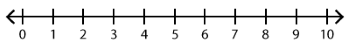##### Notes

Daily Math Practice provides a week's worth of problems ideal for morning work or review. Week 22 reviews standards from previous weeks and supports Common Core Mathematics Standards K.OA.A.1, K.OA.A.2, & K.OA.A.3.

##### Print Instructions

NOTE: Only your test content will print.
To preview this test, click on the File menu and select Print Preview.

See our guide on How To Change Browser Print Settings to customize headers and footers before printing.

# Kindergarten - Daily Math Practice - Week 22 (Kindergarten)

Print Test (Only the test content will print)
 DAILY MATH PRACTICE - WEEK 22 DAY 1 DAY 2 Subtract. Use the number line.8 - 6 = Subtract. Use the number line.10 - 5 = There are 5 muffins in a box. Lee eats 1 muffin. How many muffins are left in the box?Draw a picture:Answer:muffins Chase sees 7 cars parked on the street. When he looks later, there are only 5 cars parked on the street. How many cars left?Write a subtraction sentence:Answer:cars Circle the addition sentence for the model.3 + 3 = 61 + 5 = 6 Circle the addition sentence for the model.5 + 1 = 62 + 4 = 6 Write the number that is one more than 15.Color the shapes with 6 sides.DAILY MATH PRACTICE - WEEK 22 DAY 3 DAY 4 Subtract. Use the number line.5 - 3 = Subtract. Use the number line.6 - 6 = Best Market has 7 juice flavors. It sells out of 2 of the flavors. How many juice flavors are left?Draw a picture:Answer:juice flavors Theo has 9 kittens. He gives away 6 of the kittens. How many kitten does Theo have left?Write a subtraction sentence:Answer:kittens Circle the addition sentence for the model.3 + 3 = 65 + 1 = 6 Circle the addition sentence for the model.1 + 5 = 64 + 2 = 6 Circle the larger number.6 or 9 Write the missing number.19 = 1 ten +onesYou need to be a HelpTeaching.com member to access free printables.